# Lecturers slides http www comp nus edu sgcs

• Slides: 14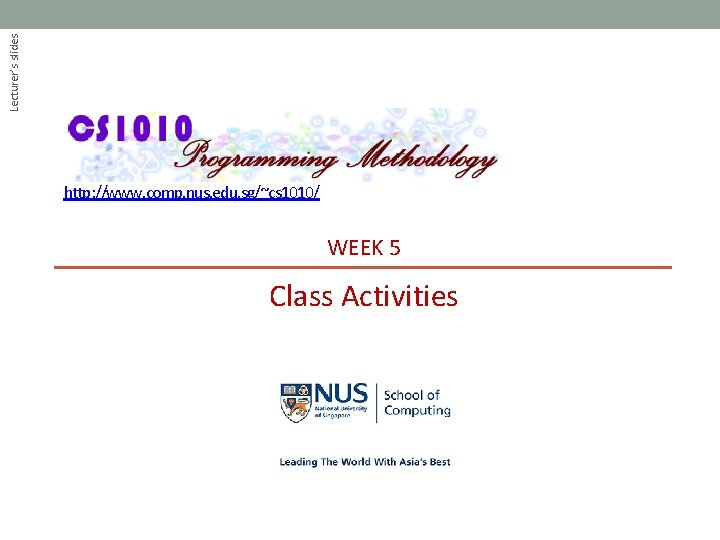Lecturer’s slides http: //www. comp. nus. edu. sg/~cs 1010/ WEEK 5 Class Activities© NUS CS 1010 (AY 2014/5 Semester 1) Week 5 - 2 Week 5: Pointers & Arrays Pointers § Tracing Pointers § Choose the Correct Codes § Incrementing a Pointer Arrays § § Exercise #1: Reversing an Array Exercise #2: Missing Digits Exercise #3: Modularising “Missing Digits” program Exercise #4: Set Containment – Take home if time runs out© NUS CS 1010 (AY 2014/5 Semester 1) Tracing Pointers (1/2) § Trace the code below manually to obtain the outputs. § Compare your outputs with your neighbours. int a = 8, b = 15, c = 23; int *p 1, *p 2, *p 3; Week 5_Trace. Pointers. c p 1 = &b; p 2 = &c; p 3 = p 2; printf("1: %d %d %dn", *p 1, *p 2, *p 3); *p 1 *= a; while (*p 2 > 0) { *p 2 -= a; (*p 1)++; } printf("2: %d %d %dn", *p 1, *p 2, *p 3); printf("3: %d %d %dn", a, b, c); Week 5 - 3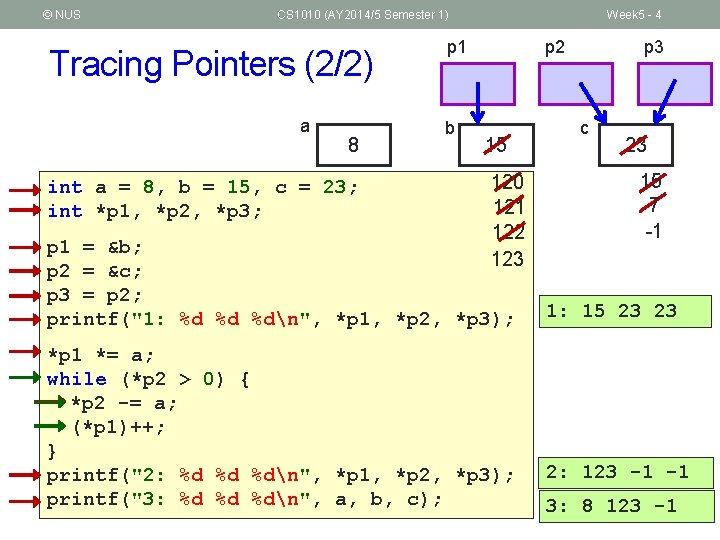© NUS CS 1010 (AY 2014/5 Semester 1) Tracing Pointers (2/2) a 8 int a = 8, b = 15, c = 23; int *p 1, *p 2, *p 3; Week 5 - 4 p 1 b p 2 15 120 121 122 123 p 1 = &b; p 2 = &c; p 3 = p 2; printf("1: %d %d %dn", *p 1, *p 2, *p 3); *p 1 *= a; while (*p 2 > 0) { *p 2 -= a; (*p 1)++; } printf("2: %d %d %dn", *p 1, *p 2, *p 3); printf("3: %d %d %dn", a, b, c); p 3 c 23 15 7 -1 1: 15 23 23 2: 123 -1 -1 3: 8 123 -1© NUS CS 1010 (AY 2014/5 Semester 1) Week 5 - 5 Choose the Correct Codes § Pick the correct codes to read a value into the float variable var. (A) (B) float var; scanf("%f", var) (C) float var; float *p; p = &var; scanf("%f", p) float var; scanf("%f", &var) (D) float var; float *p; p = &var; scanf("%f", &p)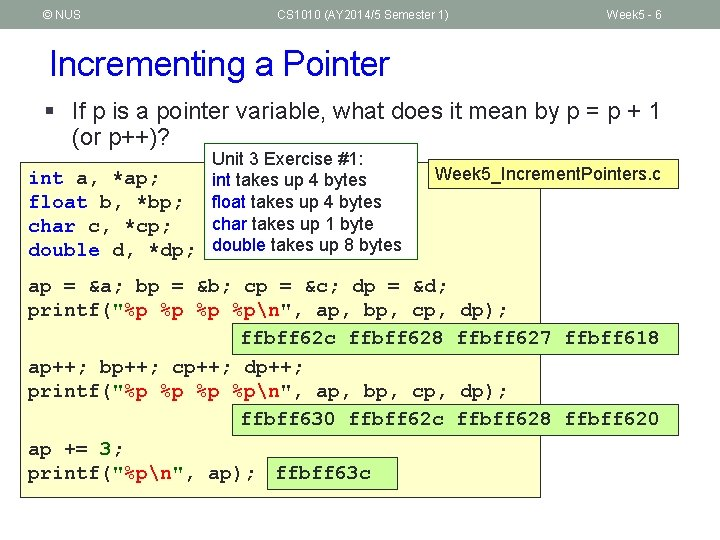© NUS CS 1010 (AY 2014/5 Semester 1) Week 5 - 6 Incrementing a Pointer § If p is a pointer variable, what does it mean by p = p + 1 (or p++)? Unit 3 Exercise #1: int a, *ap; int takes up 4 bytes float b, *bp; float takes up 4 bytes char takes up 1 byte char c, *cp; double d, *dp; double takes up 8 bytes Week 5_Increment. Pointers. c ap = &a; bp = &b; cp = &c; dp = &d; printf("%p %pn", ap, bp, cp, ffbff 62 c ffbff 628 ap++; bp++; cp++; dp++; printf("%p %pn", ap, bp, cp, ffbff 630 ffbff 62 c ap += 3; printf("%pn", ap); ffbff 63 c dp); ffbff 627 ffbff 618 dp); ffbff 628 ffbff 620© NUS CS 1010 (AY 2014/5 Semester 1) Week 5 - 7 Exercise #1: Reversing an Array § Write a program Week 5_Reverse. Array. c to read a list of numbers (at most 10 of them) into the array, reverse the array and print its elements. § We will write everything in the main() function for now. § An incomplete program Week 5_Reverse. Array. c is given. § Sample run: Enter size of array (<=10): 5 Enter 5 elements: 1 -2 3 8 6 After reversing: 6 8 3 -2 1© NUS CS 1010 (AY 2014/5 Semester 1) Week 5 - 8 Exercise #2: Missing Digits (1/3) § Write a program Week 5_Missing. Digits. c to read in a positive integer and list out all the digits that do not appear in the input number. § We will write everything in the main() function. (You will modularise it in exercise #3. ) § Sample run: Enter a number: 73015 Missing digits in 73015: 2 4 6 8 9 § Recall: How do we extract individual digits from an integer?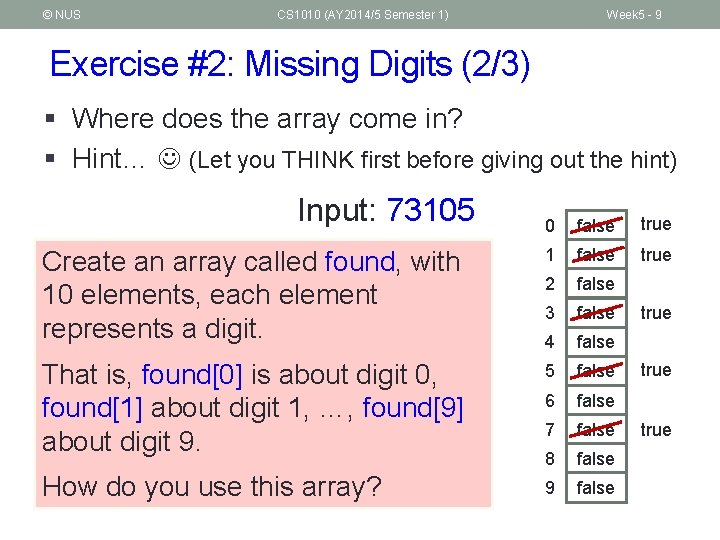© NUS CS 1010 (AY 2014/5 Semester 1) Week 5 - 9 Exercise #2: Missing Digits (2/3) § Where does the array come in? § Hint… (Let you THINK first before giving out the hint) Input: 73105 0 false true Create an array called found, with 10 elements, each element represents a digit. 1 false true 2 false 3 false 4 false That is, found is about digit 0, found about digit 1, …, found about digit 9. 5 false 6 false 7 false 8 false How do you use this array? 9 false true© NUS CS 1010 (AY 2014/5 Semester 1) Week 5 - 10 Exercise #2: Missing Digits (3/3) Show this only after students have attempted it themselves. int main(void) { int number, i; int found = {0} // found[i]=0 means digit i is missing printf("Enter a number: "); scanf("%d", &number); printf("Missing digits in %d: ", number); Key idea while (number > 0) { found[number%10] = 1; // found digit in input number /= 10; } for (i = 0; i < 10; i++) { if (!found[i]) printf("%d ", i); } printf("n"); return 0; } Week 5_Missing. Digits. c© NUS CS 1010 (AY 2014/5 Semester 1) Week 5 - 11 Exercise #3: Modularising Exercise #2 § Let’s re-write our program Week 5_Missing. Digits. c into Week 5_Missing. Digits. Modular. c § Objective: Passing array to a function § The program should contain a function called analyse. Number() that takes in a number and analyse what are the missing digits in that number § What is/are the parameter(s)? § The program should also contain a function called print. Missing. Digits() to print out all the missing digits § What is/are the parameter(s)?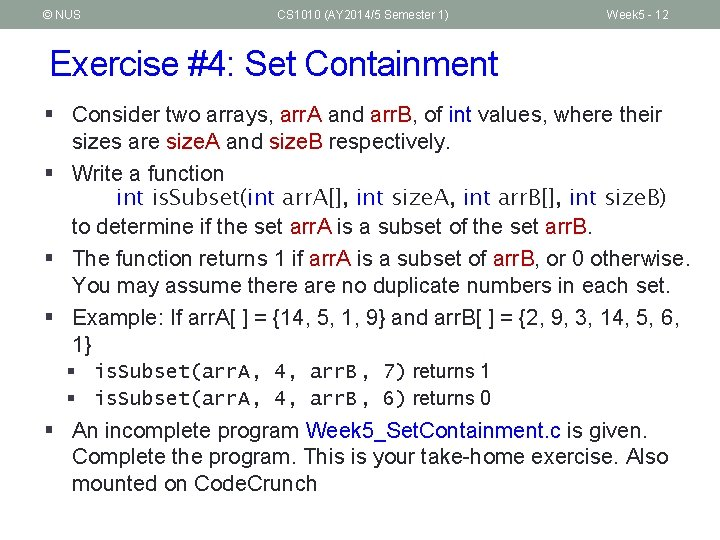© NUS CS 1010 (AY 2014/5 Semester 1) Week 5 - 12 Exercise #4: Set Containment § Consider two arrays, arr. A and arr. B, of int values, where their sizes are size. A and size. B respectively. § Write a function int is. Subset(int arr. A[], int size. A, int arr. B[], int size. B) to determine if the set arr. A is a subset of the set arr. B. § The function returns 1 if arr. A is a subset of arr. B, or 0 otherwise. You may assume there are no duplicate numbers in each set. § Example: If arr. A[ ] = {14, 5, 1, 9} and arr. B[ ] = {2, 9, 3, 14, 5, 6, 1} § is. Subset(arr. A, 4, arr. B, 7) returns 1 § is. Subset(arr. A, 4, arr. B, 6) returns 0 § An incomplete program Week 5_Set. Containment. c is given. Complete the program. This is your take-home exercise. Also mounted on Code. Crunch© NUS CS 1010 (AY 2014/5 Semester 1) Things-To-Do n Revise n n Deadline for Lab #2 n n Deadline: 13 September 2014, Saturday, 9 am Preparation for next week n n Chapter 6: Numeric Arrays Multi-dimensional arrays Continue to do practice exercises on Code. Crunch Week 5 - 13© NUS CS 1010 (AY 2014/5 Semester 1) End of File Week 5 - 14